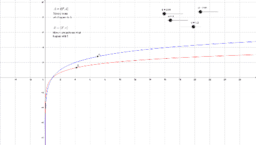# Logarithms

Author:
R. Johnson
Topic:
Logarithm
8^x=1/64 By the equations for logarithm definition we have that x=log8(1/64)=log8(1)-log8(8^2)=-2*log8(8)=-2
•### Exponential Equation

•